# Worst Value-at-Risk under Known Margins

#### 2022-05-31

library(qrmtools)
library(copula)
library(combinat) # for permn()
library(sfsmisc) # for eaxis()
doPDF <- FALSE

## 1 Homogeneous case

We start by considering the following setup in the homogeneous case, that is, when all marginal distributions are equal.

qF2 <- function(p, th = 2) qPar(p, shape = th) # Par(2) quantile function
pF2 <- function(q, th = 2) pPar(q, shape = th) # Par(2) distribution function
dim <- 8 # variable dimension (we use 8 or 100 here)

### 1.1 Checks for method = “dual”

Investigate the helper function $$h(s,t)$$ (the function for the inner root-finding to compute $$D(s)$$; see dual_bound()).

d <- 8 # dimension
s <- c(1, 5, 10, 100, 500, 1000)
t <- sapply(seq_along(s), function(i) {
res <- exp(seq(log(1e-3), log(s[i]/d), length.out = 257))
res[length(res)] <- s[i]/d # to avoid numerical issues (t > s/d)
res
})
f <- sapply(seq_along(s), function(i)
sapply(t[,i], function(t.)
qrmtools:::dual_bound_2(s[i], t = t., d = d, pF = pF2) -
qrmtools:::dual_bound_2_deriv_term(s[i], t = t., d = d, pF = pF2)))
palette <- colorRampPalette(c("maroon3", "darkorange2", "royalblue3"), space = "Lab")
cols <- palette(6)
if(doPDF)
pdf(file = (file <- paste0("fig_worst_VaR_hom_dual_h_Par=2_d=",d,".pdf")),
width = 6, height = 6)
plot(t[,1], f[,1], type = "l", log = "x", xlim = range(t), ylim = range(f), col = cols,
xlab = "t", ylab = expression("h(s,t) for d = 8 and F being Par(2)"))
lines(t[,2], f[,2], col = cols)
lines(t[,3], f[,3], col = cols)
lines(t[,4], f[,4], col = cols)
lines(t[,5], f[,5], col = cols)
lines(t[,6], f[,6], col = cols)
abline(h = 0, lty = 2)
legend("topright", lty = rep(1,6), col = cols,
bty = "n", legend = as.expression(lapply(1:6,
function(i) substitute(s==s., list(s. = s[i])))))
if(doPDF) dev.off()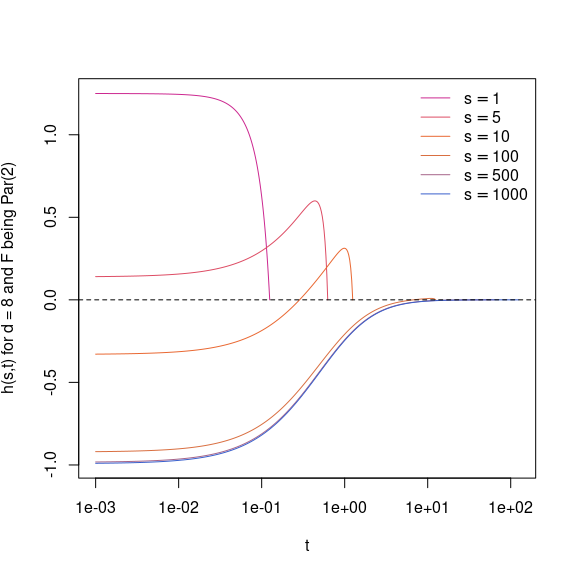As we know, $$h(s,s/d) = 0$$. We also see that $$s$$ has to be sufficiently large in order to find a root $$h(s,t) = 0$$ for $$t < s/d$$.

Now let’s plot the dual bound $$D(s)$$ for various $$\theta$$ (this checks the outer root-finding).

theta <- c(0.5, 1, 2, 4) # theta values
s <- seq(48, 2000, length.out = 257) # s values
D <- sapply(theta, function(th)
sapply(s, function(s.)
dual_bound(s., d = d, pF = function(q) pPar(q, shape = th)))) # (s, theta) matrix
if(doPDF)
pdf(file = (file <- paste0("fig_worst_VaR_hom_dual_D_s_Par=",
paste0(theta, collapse="_"),"_d=",d,".pdf")),
width = 6, height = 6)
plot(s, D[,1], type = "l", ylim = range(D), col = "maroon3",
ylab = substitute("Dual bound D(s) for d ="~d.~"Par("*theta*") margins", list(d. = d)))
lines(s, D[,2], col = "darkorange2")
lines(s, D[,3], col = "royalblue3")
lines(s, D[,4], col = "black")
legend("topright", lty = rep(1,4),
col = c("maroon3", "darkorange2", "royalblue3", "black"),
bty = "n", legend = as.expression(lapply(1:4,
function(j) substitute(theta==j, list(j = theta[j])))))
if(doPDF) dev.off()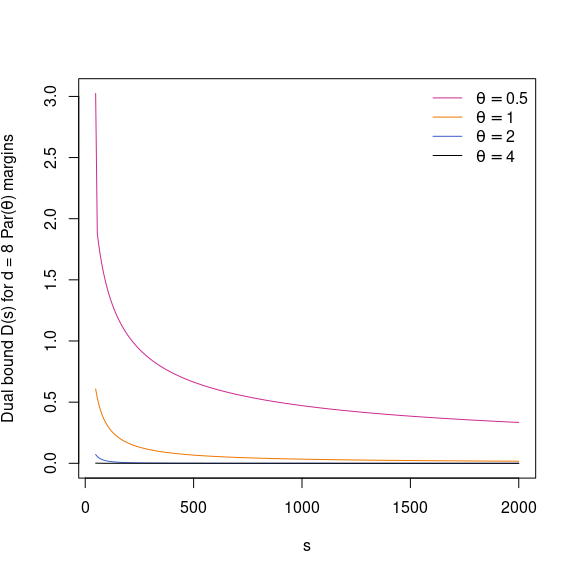### 1.2 Checks for method = “Wang”/“Wang.Par”

#### 1.2.1 Check of auxiliary functions with numerical integration (for $$\theta = 2$$)

Check Wang_h_aux().

d <- 8 # dimension
alpha <- 0.99 # confidence level
c <- seq(0, (1-alpha)/d, length.out = 129) # domain of h
h.aux <- qrmtools:::Wang_h_aux(c, level = alpha, d = d, qF = qF2)
par(mar = c(5, 4+1, 4, 2) + 0.1) # increase space (for y axis label)
plot(c, h.aux, type = "l", xlab = "c (in initial interval)",
ylab = expression(frac(d-1,d)~{F^{-1}}(a[c])+frac(1,d)~{F^{-1}}(b[c])))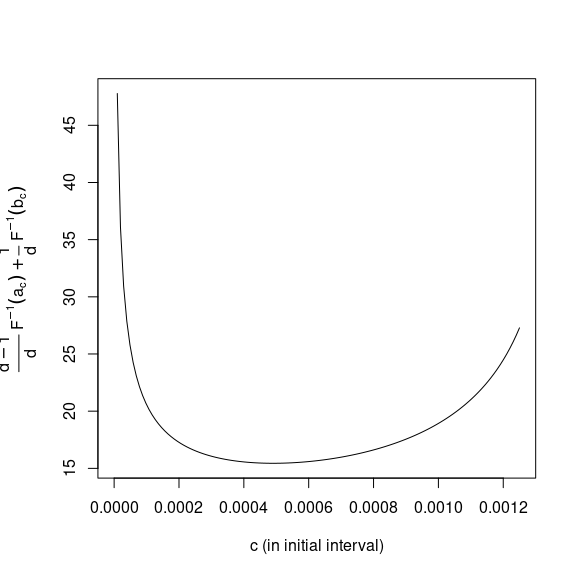Check the objective function $$h(c)$$ (that is, Wang_h() with numerical integration) on its domain. Note that $$h$$ is used to determine the root in the open interval $$(0,(1-\alpha)/d)$$ for computing worst value-at-risk.

h <- sapply(c, function(c.) qrmtools:::Wang_h(c., level = alpha, d = d, qF = qF2))
if(doPDF)
pdf(file = (file <- paste0("fig_worst_VaR_",alpha,"_hom_Wang_h_Par=2_d=",d,"_num.pdf")),
width = 6, height = 6)
plot(c, h, type = "l", xlab = "c (in initial interval)",
ylab = substitute("h(c) for"~~alpha~"= 0.99 and d ="~d.~"Par(2) margins",
list(d. = d)))
abline(h = 0, lty = 2)
if(doPDF) dev.off()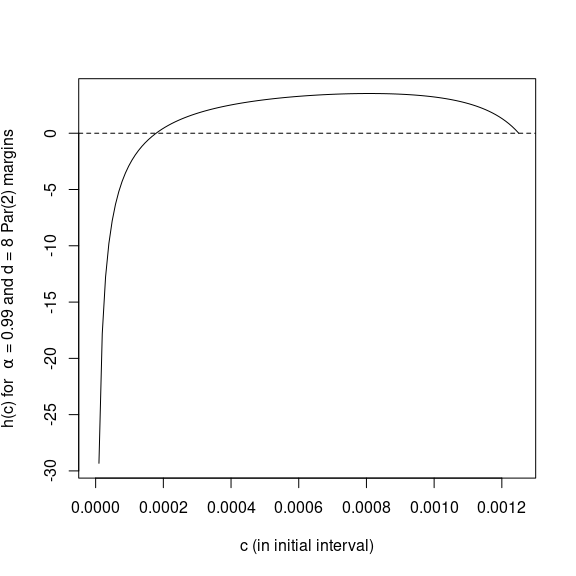Check the objective function $$h$$ at the endpoints of its domain.

sapply(c(0, (1-alpha)/d), function(c.)
qrmtools:::Wang_h(c., level = alpha, d = d, qF = qF2)) # -Inf, 0
##  -Inf    0

$$-\infty$$ is not a problem for root finding (for $$\theta > 1$$; for $$\theta <= 1$$ it is NaN, see below, and thus a problem!), but the $$0$$ at the right endpoint is a problem.

method <- "Wang.Par" # this also holds for (the numerical) method = "Wang"
th <- 0.99
qrmtools:::Wang_h(0, level = alpha, d = d, method = method, shape = th) # NaN => uniroot() fails
##  NaN
## Note: Wang_h() is actually already NaN for c <= 1e-17
qrmtools:::Wang_h_aux(0, level = alpha, d = d, method = method, shape = th) # Inf
##  Inf

A proper initial interval $$[c_l,u_l]$$ with $$0<c_l<=c_u<(1-\alpha)/d$$ (containing the root) is thus required. We have derived this in Hofert et al. (2017, Improved Algorithms for Computing Worst Value-at-Risk’’)).

#### 1.2.2 Check of $$h(c)$$without numerical integration (for a range of $$\theta$$)

Check the objective function $$h(c)$$ (Wang_h() without numerical integration)

d <- dim # dimension
alpha <- 0.99 # confidence level
theta <- c(0.1, 0.5, 1, 5, 10, 50) # theta values
palette <- colorRampPalette(c("darkorange2", "maroon3", "royalblue3", "black"), space = "Lab")
cols <- palette(length(theta))
c <- seq(0, (1-alpha)/d, length.out = 2^13+1)
## => They all go further down to 0 if length.out is increased.
##    Smaller theta thus corresponds to a larger derivative in the root
##    Root-finding thus requires higher precision for smaller theta
h <- matrix(, nrow = length(c), ncol = length(theta))
for(j in 1:length(theta))
h[,j] <- sapply(c, function(c.)
qrmtools:::Wang_h(c., level = alpha, d = d, method = "Wang.Par", shape = theta[j]))
z <- h
z[z <= 0] <- NA # > 0 => makes log-scale possible
if(doPDF)
pdf(file = (file <- paste0("fig_worst_VaR_",alpha,"_hom_Wang_h_Par_d=",d,".pdf")),
width = 6, height = 6)
plot(NA, xlim = range(c), ylim = range(z, na.rm = TRUE), log = "y", yaxt = "n", xlab = "c",
ylab = substitute("h(c) for"~~alpha~"= 0.99, d ="~d.~"Par("*theta*") margins",
list(d. = d)))
eaxis(2)
for(k in 1:length(theta))
lines(c, z[,k], col = cols[k])
legend("topleft", bty = "n", lty = rep(1,length(theta)), col = cols,
legend = as.expression(lapply(1:length(theta),
function(k) substitute(theta==k, list(k = theta[k])))))
if(doPDF) dev.off()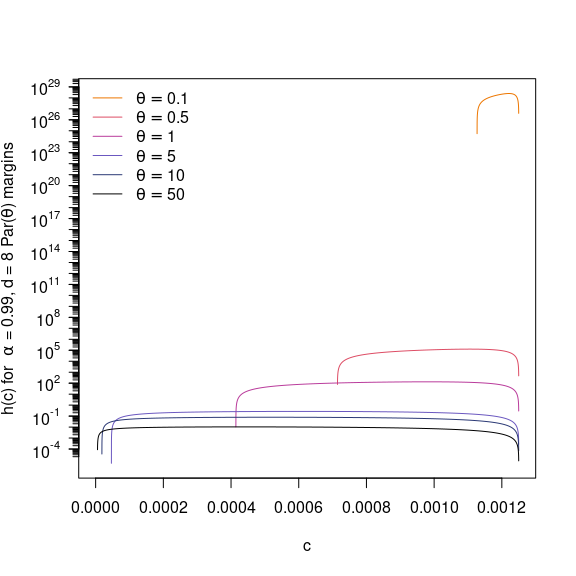### 1.3 Compute best/worst $$\mathrm{VaR}_\alpha$$ (via “Wang.Par”)

After dealing with various numerical issues, we can now look at some example calculations of best/worst value-at-risk. We first plot value-at-risk as a function of $$\alpha$$.

d <- dim # dimension
alpha <- 1-2^seq(-0.001, -10, length.out = 128) # confidence levels; concentrated near 1
theta <- c(0.1, 0.5, 1, 5, 10, 50) # theta values
VaR <- simplify2array(sapply(alpha, function(a)
sapply(theta, function(th) VaR_bounds_hom(a, d = d, method = "Wang.Par",
shape = th)), simplify = FALSE))
## => (best/worst VaR, theta, alpha)-matrix
if(doPDF)
pdf(file = (file <- paste0("fig_worst_VaR_hom_Wang_Par_d=",d,".pdf")),
width = 7, height = 7)
par(mar = c(5, 4+1, 4, 2) + 0.1) # increase space (for y axis label)
plot(NA, xlim = range(alpha), ylim = range(VaR), log = "xy", yaxt = "n",
xlab = expression(1-alpha),
ylab = as.expression(substitute(underline(VaR)[alpha](L^{"+"})~"(dashed) and"~bar(VaR)[alpha](L^{"+"})~
"(solid) for d ="~d.~"and Par("*theta*") margins",
list(d. = d))))
eaxis(2)
for(k in 1:length(theta)) {
lines(1-alpha, VaR[2,k,], col = cols[k]) # worst VaR
lines(1-alpha, VaR[1,k,], col = cols[k], lty = 2) # best VaR
}
legend("topright", bty = "n", lty = rep(1,length(theta)), col = cols,
legend = as.expression(lapply(1:length(theta),
function(k) substitute(theta==k, list(k = theta[k])))))
if(doPDF) dev.off()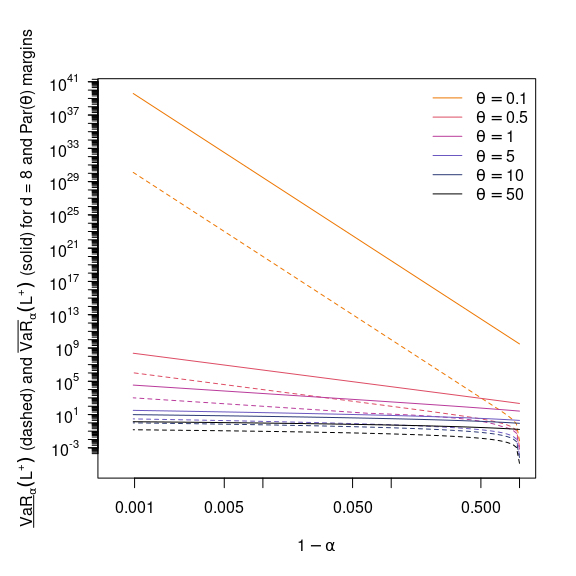Now consider best/worst value-at-risk as a function of $$d$$.

d <- seq(2, 1002, by = 20) # dimensions
alpha <- 0.99 # confidence level
theta <- c(0.1, 0.5, 1, 5, 10, 50) # theta values
VaR <- simplify2array(sapply(d, function(d.)
sapply(theta, function(th) VaR_bounds_hom(alpha, d = d., method = "Wang.Par",
shape = th)), simplify = FALSE))
## => (best/worst VaR, theta, d)-matrix
if(doPDF)
pdf(file = (file <- paste0("fig_worst_VaR_hom_Wang_Par_alpha=",alpha,"_in_d.pdf")),
width = 7, height = 7)
par(mar = c(5, 4+1, 4, 2) + 0.1) # increase space (for y axis label)
plot(NA, xlim = range(d), ylim = range(VaR), log = "xy", yaxt = "n",
xlab = expression(d),
ylab = as.expression(substitute(underline(VaR)[a](L^{"+"})~"(dashed) and"~bar(VaR)[a](L^{"+"})~
"(solid) for Par("*theta*") margins",
list(a = alpha))))
eaxis(2)
for(k in 1:length(theta)) {
lines(d, VaR[2,k,], col = cols[k]) # worst VaR
lines(d, VaR[1,k,], col = cols[k], lty = 2) # best VaR
}
legend("topleft", bty = "n", lty = rep(1,length(theta)), col = cols,
legend = as.expression(lapply(1:length(theta),
function(k) substitute(theta==k, list(k = theta[k])))))
if(doPDF) dev.off()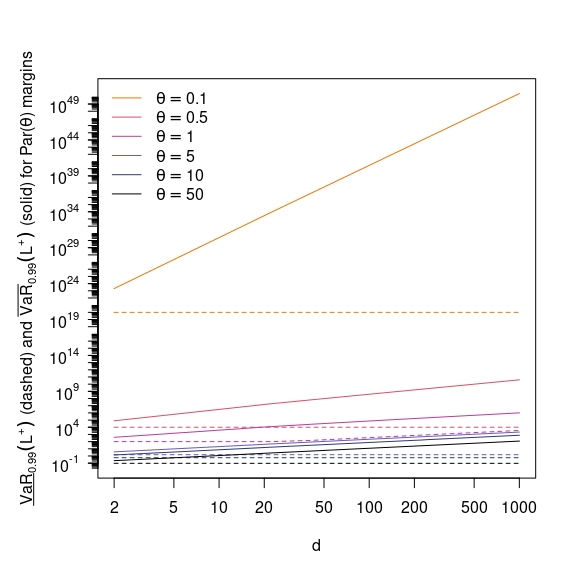We can also consider best/worst value-at-risk as a function of $$\theta$$. Note that, as before, best value-at-risk is the same for all $$d$$ here (depicted in a green dashed line below).

d <- c(2, 10, 20, 100, 200, 1000) # dimensions
theta <- 10^seq(-1, log(50, base = 10), length.out = 50) # theta values
alpha <- 0.99 # confidence level
VaR <- simplify2array(sapply(d, function(d.)
sapply(theta, function(th) VaR_bounds_hom(alpha, d = d., method = "Wang.Par",
shape = th)), simplify = FALSE))
## => (best/worst VaR, theta, d)-matrix
if(doPDF)
pdf(file = (file <- paste0("fig_worst_VaR_hom_Wang_Par_alpha=",alpha,"_in_theta.pdf")),
width = 6, height = 6)
par(mar = c(5, 4+1, 4, 2) + 0.1) # increase space (for y axis label)
plot(NA, xlim = range(theta), ylim = range(VaR), log = "xy", yaxt = "n",
xlab = expression(theta),
ylab = as.expression(substitute(underline(VaR)[a](L^{"+"})~"(dashed) and"~
bar(VaR)[a](L^{"+"})~"(solid) for Par("*theta*") margins",
list(a = alpha))))
eaxis(2)
bestVaR <- VaR[1,,1]
for(k in 1:length(d)) {
lines(theta, VaR[2,,k], col = cols[k]) # worst VaR
if(k >= 2) stopifnot(all.equal(bestVaR, VaR[1,,k]))
}
lines(theta, bestVaR, col = "darkgreen", lty = 2) # best VaR
legend("topright", bty = "n", lty = rep(1,length(d)), col = cols,
legend = as.expression(lapply(1:length(d),
function(k) substitute(d==k, list(k = d[k])))))
if(doPDF) dev.off()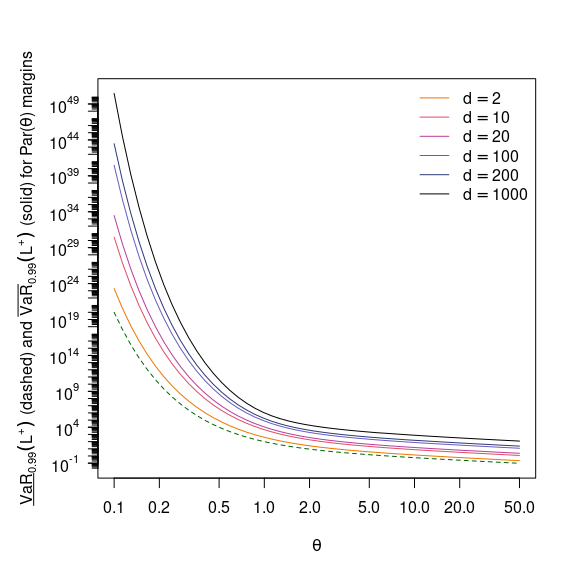### 1.4 Comparison between various methods for computing worst value-at-risk

Now consider a graphical comparison between the various methods. To stress the numerical challenges, let us include the following implementation of Wang’s approach (with various switches to highlight the individual numerical challenges) for the Pareto case.

## Initial interval for the root finding in case of worst VaR
init_interval <- function(alpha, d, shape, trafo = FALSE, adjusted = FALSE)
{
if(trafo) {
low <- if(shape == 1) {
d/2
} else {
(d-1)*(1+shape)/(d-1+shape)
}
up <- if(shape > 1) {
r <- (1+d/(shape-1))^shape
} else if(shape == 1) {
e <- exp(1)
(d+1)^(e/(e-1))
} else {
d*shape/(1-shape)+1
}
c(low, up)
} else {
low <- if(shape > 1) {
r <- (1-alpha)/((d/(shape-1)+1)^shape + d-1)
} else if(shape == 1) {
e <- exp(1)
(1-alpha)/((d+1)^(e/(e-1))+d-1)
} else {
r <- (1-shape)*(1-alpha)/d
}
up <- if(shape == 1) (1-alpha)/(3*d/2-1)
else (1-alpha)*(d-1+shape)/((d-1)*(2*shape+d))
c(low, up)
}
}

## Function to compute the best/worst value-at-risk in the homogeneous case with
## Par(theta) margins
VaR_hom_Par <- function(alpha, d, shape, method = c("worst", "best"),
trafo = FALSE, interval = NULL, adjusted = FALSE,
avoid.cancellation = FALSE, ...)
{
## Pareto quantile function
qF <- function(p) (1 - p)^(-1/shape) - 1

## Compute \bar{I}
Ibar <- function(a, b, alpha, d, shape)
{
if(shape == 1) log((1-a)/(1-b))/(b-a) - 1
else (shape/(1-shape))*((1-b)^(1-1/shape)-(1-a)^(1-1/shape))/(b-a) - 1
}

## Main
method <- match.arg(method)
switch(method,
"worst" = {

## Distinguish according to whether we optimize the auxiliary function
## on a transformed scale
h <- if(trafo) {
## Auxiliary function to find the root of on (1, Inf)
if(shape == 1) {
function(x) x^2 + x*(-d*log(x)+d-2)-(d-1)
} else {
function(x)
(d/(1-shape)-1)*x^(-1/shape + 1) - (d-1)*x^(-1/shape) + x - (d*shape/(1-shape) + 1)
}
} else {
## Auxiliary function to find the root of on (0, (1-alpha)/d)
function(c) {
a <- alpha+(d-1)*c
b <- 1-c
Ib <- if(c == (1-alpha)/d) { # Properly deal with limit c = (1-alpha)/d
((1-alpha)/d)^(-1/shape) - 1
} else {
Ibar(a = a, b = b, alpha = alpha, d = d, shape = shape)
}
Ib - (qF(a)*(d-1)/d + qF(b)/d)
}
}

## Do the optimization
if(is.null(interval)) interval <- init_interval(alpha, d, shape,
c <- uniroot(h, interval = interval, ...)$root if(trafo) # convert value back to the right scale (c-scale) c <- (1-alpha)/(c+d-1) if(avoid.cancellation) { t1 <- (1-alpha)/c-(d-1) d * ((c^(-1/shape)/d) * ((d-1)*t1^(-1/shape) + 1) - 1) # = qF(a)*(d-1) + qF(b) } else { a <- alpha+(d-1)*c b <- 1-c qF(a)*(d-1) + qF(b) } }, "best" = { max((d-1)*0 + (1-alpha)^(-1/shape)-1, # Note: Typo in Wang, Peng, Yang (2013) d*Ibar(a = 0, b = alpha, alpha = alpha, d = d, shape)) }, stop("Wrong 'method'")) } For the comparison, consider the following setup. alpha <- 0.99 # confidence level d <- dim # dimension n.th <- 32 # number of thetas th <- seq(0.2, 5, length.out = n.th) # thetas qFs <- lapply(th, function(th.) {th.; function(p) qPar(p, shape = th.)}) # n.th-vector of Pareto quantile functions pFs <- lapply(th, function(th.) {th.; function(q) pPar(q, shape = th.)}) # n.th-vector of Pareto dfs N <- 1e4 # number of discretization points for RA(); N = 1e5 does not improve the situation Now compute the values and plot them. res <- matrix(, nrow = n.th, ncol = 7) colnames(res) <- c("Wang", "straightforward", "transformed", "Wang.Par", "dual", "RA.low", "RA.up") pb <- txtProgressBar(max = n.th, style = if(isatty(stdout())) 3 else 1) # setup progress bar for(i in seq_len(n.th)) { ## "Wang" (numerical integration with smaller uniroot() tolerance; still ## numerically critical -- we catch "the integral is probably divergent"-errors here) Wang.num.res <- tryCatch(VaR_bounds_hom(alpha, d = d, qF = qFs[[i]]), error = function(e) e) res[i,"Wang"] <- if(is(Wang.num.res, "simpleError")) NA else Wang.num.res ## Our straightforward implementation res[i,"straightforward"] <- VaR_hom_Par(alpha, d = d, shape = th[i]) ## Our straightforward implementation based on the transformed auxiliary function res[i,"transformed"] <- VaR_hom_Par(alpha, d = d, shape = th[i], trafo = TRUE) ## "Wang.Par" (using a smaller uniroot() tolerance and adjusted initial interval) res[i,"Wang.Par"] <- VaR_bounds_hom(alpha, d = d, method = "Wang.Par", shape = th[i]) ## "dual" (with uniroot()'s default tolerance) res[i,"dual"] <- VaR_bounds_hom(alpha, d = d, method = "dual", interval = crude_VaR_bounds(alpha, qF = qFs[[i]], d = d), pF = pFs[[i]]) ## Rearrangement Algorithm set.seed(271) # use the same random permutation for each theta RA. <- RA(alpha, qF = rep(qFs[i], d), N = N) res[i,"RA.low"] <- RA.$bounds
res[i,"RA.up"]  <- RA.$bounds ## Progress setTxtProgressBar(pb, i) # update progress bar } close(pb) # close progress bar res. <- res/res[,"dual"] # standardize (by dual method) ylim <- range(res., na.rm = TRUE) if(doPDF) pdf(file = (file <- paste0("fig_worst_VaR_",alpha,"_hom_comparison_d=", d,"_N=",N,".pdf")), width = 7, height = 7) par(mar = c(5, 4+1, 4, 2) + 0.1) # increase space (for y axis label) plot(th, res.[,"Wang"], type = "l", ylim = ylim, xlab = expression(theta), ylab = substitute("Standardized (by dual method)"~ bar(VaR)[0.99]~"for d ="~d.~"Par("*theta*") margins", list(d. = d)), col = "gray60", lty = 2, lwd = 5.5) # Wang (with numerical integration) lines(th, res.[,"straightforward"], col = "maroon3", lty = 1, lwd = 1) # still bad (although we have an initial interval) lines(th, res.[,"transformed"], col = "black", lty = 1, lwd = 1) # okay lines(th, res.[,"Wang.Par"], col = "royalblue3", lty = 2, lwd = 2.5) # Wang Pareto (wo num. integration) lines(th, res.[,"RA.low"], col = "black", lty = 3, lwd = 1) # lower RA bound lines(th, res.[,"RA.up"], col = "black", lty = 2, lwd = 1) # upper RA bound legend("topright", bty = "n", col = c("gray60", "maroon3", "black", "royalblue3", "black", "black"), lty = c(2,1,1,2,3,2), lwd = c(5.5,1,1,2.5,1,1), legend = c("Wang (num. int.)", "Wang Pareto (straightforward)", "Wang Pareto (transformed)", "Wang Pareto (wo num. int.)", "Lower RA bound", "Upper RA bound")) if(doPDF) dev.off()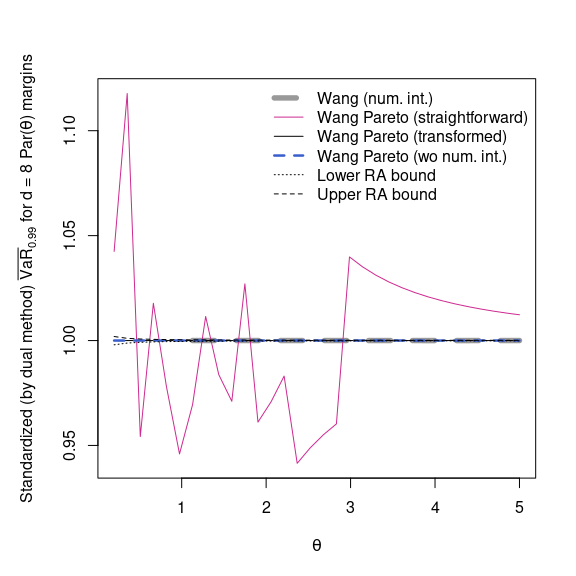So what goes wrong with our straightforward implementation? Let’s start with the obvious. As we can infer from the x-axis scale in the plots of $$h$$ above, uniroot()’s default tolerance tol = .Machine$double.eps^0.25($$\approx 0.0001220703$$ here) is too large to determine the root accurately. In fact, if we choose a smaller tol, we have no problem.

tol <- 2.2204e-16
wVaR.tol <- sapply(th, function(th.)
VaR_hom_Par(alpha = alpha, d = d, shape = th., tol = tol))
plot(th, wVaR.tol/res[,"dual"], type = "l", ylim = ylim,
xlab = expression(theta), ylab = "Wang's approach (straightforward) but with smaller tol")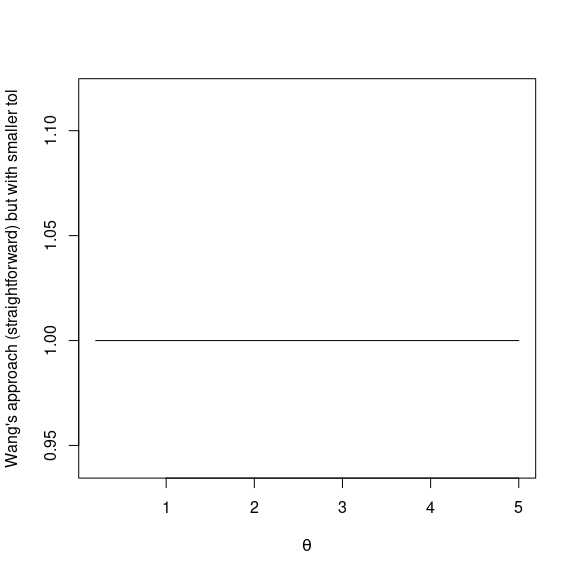So is this the solution to all the problems? No. Consider the setup as before, where $$d$$ is running (we catch the appearing errors here).

alpha <- 0.99 # confidence level
d <- seq(2, 1002, by = 20) # dimensions
theta <- c(0.1, 0.5, 1, 5, 10, 50) # theta values
VaR <- simplify2array(sapply(d, function(d.)
sapply(theta, function(th) {
res <- tryCatch(VaR_hom_Par(alpha, d = d., shape = th, tol = tol),
error = function(e) e)
if(is(res, "simpleError")) { warning(conditionMessage(res)); NA }
else res
}), simplify = FALSE))

By using warnings() we see that we cannot even determine the root in all cases as the function values are numerically not of opposite sign at the endpoints of the theoretically correct initial interval containing the root. We can solve this (non-elegantly) by simply adjusting the lower bound.

VaR <- simplify2array(sapply(d, function(d.)
sapply(theta, function(th) VaR_hom_Par(alpha, d = d., shape = th, tol = tol, adjusted = TRUE)),
simplify = FALSE))

So we see that we need a smaller tolerance and to extend the initial interval in order to get reasonable results.

What about using the transformed auxiliary function directly? Is this a numerically more stable approach?

d <- 500
th <- 20
VaR_hom_Par(alpha, d = d, shape = th, trafo = TRUE) # Inf
##  Inf

As we can see, there is a problem as well. To understand it, consider the following minimized version of VaR_hom_Par() (for computing worst value-at-risk for $$\theta\neq 1$$ only).

h <- function(x)
(d/(1-th)-1)*x^(-1/th + 1) - (d-1)*x^(-1/th) + x - (d*th/(1-th) + 1)
interval <- init_interval(alpha, d, th, trafo = TRUE)
x <- uniroot(h, interval = interval)$root (c <- (1-alpha)/(x+d-1)) # convert back to c-scale ##  1.869497e-31 a <- alpha+(d-1)*c b <- 1-c qPar(a, shape = th)*(d-1) + qPar(b, shape = th) # Inf ##  Inf stopifnot(b == 1) # => b is 1 => qPar(b, shape = th) = Inf As we can see, $$c>0$$ is so small that $$b = 1-c$$ is numerically equal to 1 and thus the Pareto quantile evaluated as $$\infty$$. This cancellation can be avoided by simplifying the terms (note that qPar(1-c, shape = th) = c^(-1/th)-1). qPar(a, shape = th)*(d-1) + c^(-1/th)-1 ##  162.5923 We can apply this to the variable combinations as before. Note that due to the partly extreme values of $$d$$ and $$\theta$$, we also need to adjust the range here in order for a root to be found. d <- seq(2, 1002, by = 20) # dimensions theta <- c(0.1, 0.5, 1, 5, 10, 50) # theta values VaR. <- simplify2array(sapply(d, function(d.) sapply(theta, function(th) VaR_hom_Par(alpha, d = d., shape = th, trafo = TRUE, adjusted = TRUE, avoid.cancellation = TRUE)), simplify = FALSE)) We can now compare the values (based on the non-transformed/transformed auxiliary function) in a graph. ylim <- range(VaR, VaR.) if(doPDF) pdf(file = (file <- paste0("fig_worst_VaR_",alpha,"_hom_comparison_num_problems.pdf")), width = 7, height = 7) par(mar = c(5, 4+1, 4, 2) + 0.1) # increase space (for y axis label) plot(d, VaR[1,], type = "l", ylim = ylim, log = "y", yaxt = "n", xlab = "d", ylab = as.expression(substitute(bar(VaR)[a](L^{"+"})~ "directly (solid) or with transformed h (dashed) for Par("*theta*") margins", list(a = alpha))), col = cols) eaxis(2) for(k in 2:length(theta)) lines(d, VaR [k,], col = cols[k]) for(k in 1:length(theta)) lines(d, VaR.[k,], col = cols[k], lty = 2, lwd = 2) legend("right", bty = "n", col = cols, lty = rep(1, length(theta)), legend = as.expression( lapply(1:length(theta), function(k) substitute(theta==th, list(th = theta[k]))) )) if(doPDF) dev.off()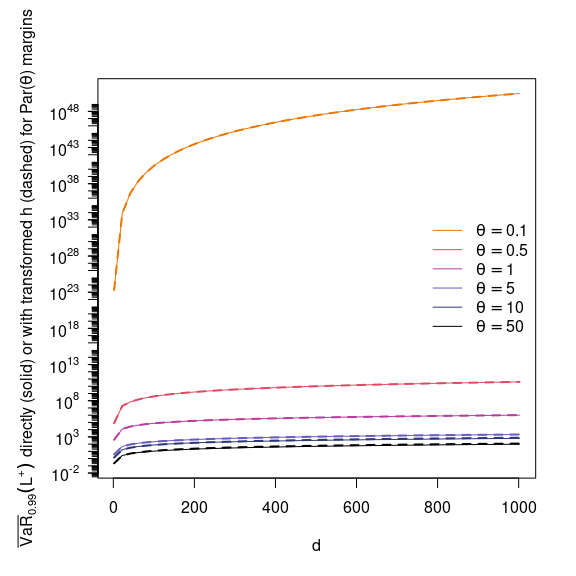We see that the results differ slightly if both $$d$$ and $$\theta$$ are large. It remains numerically challenging to appropriately compute worst value-at-risk in this case. However, both of the above approaches seem to work for dimensions even as large as $$10^5$$. ## 2 Inhomogeneous case ### 2.1 A motivation for (column) rearrangements The basic idea goes back to Iman and Conover (1982, “A distribution-free approach to inducing rank correlation among input variables”). Suppose we have 500 observations of two Pareto distributions. This is the joint sample under independence (as we independently draw the uniforms when constructing X): ## Generate samples n <- 1000 # sample size set.seed(271) # set a seed (for reproducibility) X <- cbind(qPar(runif(n), shape = 2), # Par(2) sample qPar(runif(n), shape = 2.5)) # Par(2.5) sample ## Plot if(doPDF) pdf(file = (file <- paste0("fig_RA_motivation_indep.pdf")), width = 6, height = 6) plot(X, xlab = expression(X), ylab = expression(X)) if(doPDF) dev.off()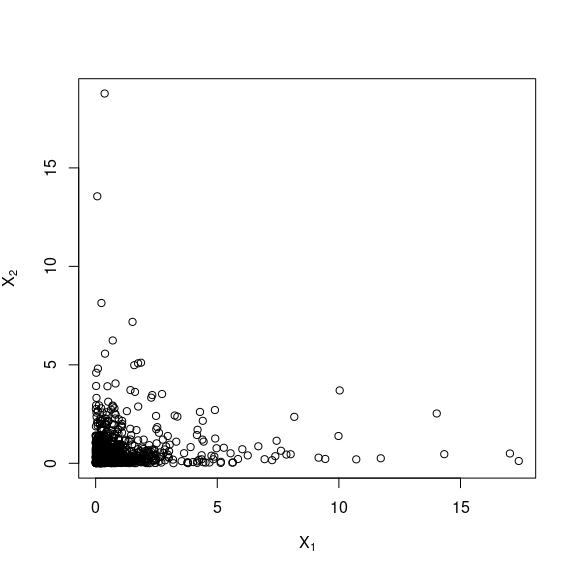If we order the realizations in each column to be in increasing order (so the smallest (largest) entry of the first column lies next to the smallest (largest) entry of the second column of X), we obtain the following (comonotone) sample. ## Generate samples X. <- apply(X, 2, sort) ## Plot if(doPDF) pdf(file = (file <- paste0("fig_RA_motivation_comonotone.pdf")), width = 6, height = 6) plot(X., xlab = expression(X), ylab = expression(X)) if(doPDF) dev.off()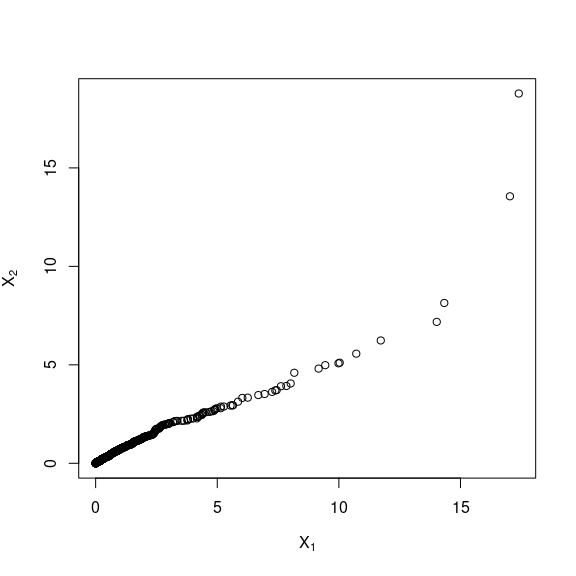We take away that column rearrangements don’t change the marginal distributions but the dependence between the two columns and thus the distributions of their sums. To see the latter (in terms of boxplots and kernel density estimates), consider: ## Samples of the sum of the components ## Note: Their mean is the same (unaffected by the dependence) Xsum <- rowSums(X) Xsum. <- rowSums(X.) ## Quick plot boxplot(Xsum, Xsum., log = "y", names = c("Independence", "Comonotonicity")) ## Kernel density estimates ran <- range(X, X.) dXsum <- density(Xsum, from = ran, to = ran) dXsum. <- density(Xsum., from = ran, to = ran) ## Plot if(doPDF) pdf(file = (file <- paste0("fig_RA_motivation_kernel_density_estimates.pdf")), width = 6, height = 6) plot(dXsum, xlim = c(0, 5), ylim = c(0, max(dXsum$y, dXsum.$y)), xlab = "x", ylab = "Kernel density estimate at x", main = "", type = "l") lines(dXsum., col = "royalblue3") legend("topright", bty = "n", lty = 1, col = c("black", "royalblue3"), legend = c("Independence", "Comonotonicity")) if(doPDF) dev.off()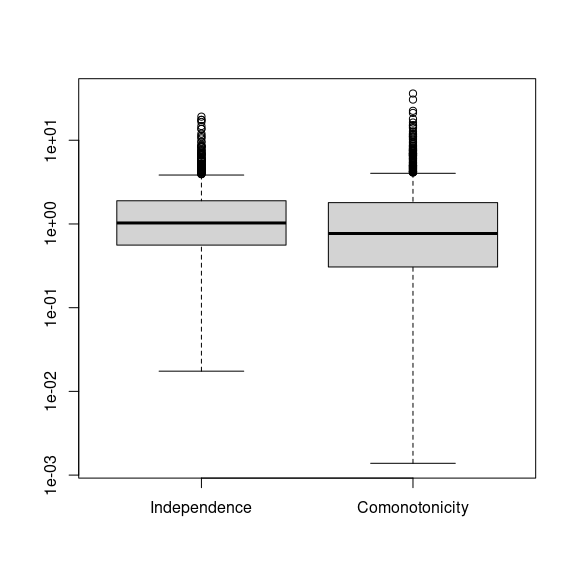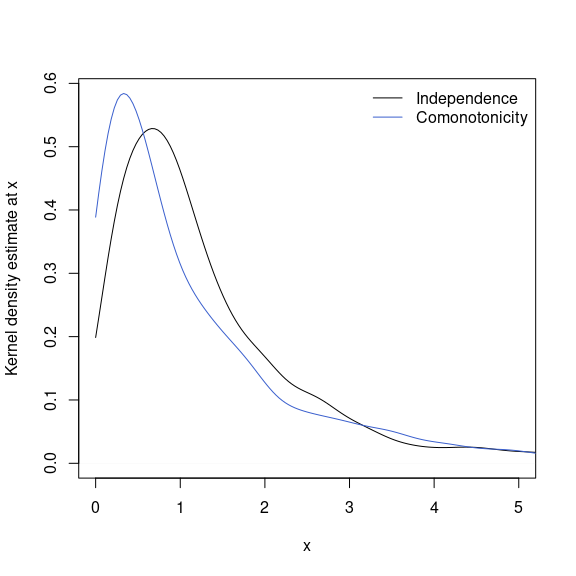### 2.2 Run-time comparison (straightforward vs efficient implementation) A straightforward (but inefficient) implementation of a basic rearrange(, sample = FALSE, is.sorted = TRUE) is the following. Note that our implementation in qrmtools allows for much greater functionality and is faster. basic_rearrange_worst_VaR <- function(X, tol = 0) { N <- nrow(X) d <- ncol(X) m.rs.old <- min(rowSums(X)) Y <- X while (TRUE) { for(j in 1:d) Y[,j] <- sort(Y[,j], decreasing = TRUE)[rank(rowSums(Y[,-j, drop = FALSE]), ties.method = "first")] Y.rs <- rowSums(Y) m.rs.new <- min(Y.rs) tol. <- abs((m.rs.new - m.rs.old)/m.rs.old) tol.reached <- if(is.null(tol)) { identical(Y, X) } else { tol. <= tol } if(tol.reached) { break } else { m.rs.old <- m.rs.new X <- Y } } min(rowSums(Y)) } We now compare this to the actual implementation (rearrange(, sample = FALSE, is.sorted = TRUE)). To this end, we consider the following setup. ## Build the input matrix (for worst VaR) alpha <- 0.99 # confidence level N <- 2^9 # number of discretization points; 512 here p <- alpha + (1-alpha)*0:(N-1)/N # probabilities for evaluating marginal quantile functions if(FALSE) d <- 2^(4:10) # 16, ..., 1024 d <- 2^(4:8) # dimensions 16, ..., 256 (to save time here) res <- matrix(, nrow = length(d), ncol = 2) # matrix containing run time results colnames(res) <- c("basic", "sophisticated") ## For each d, measure the run time (some randomness involved here...) for(i in seq_along(d)) { mar <- rep(list(qF2), d[i]) # marginal quantile functions X <- sapply(mar, function(qF) qF(p)) # evaluate marginal quantile functions at p res[i,] <- c(system.time(basic_rearrange_worst_VaR(X))[["elapsed"]], # basic version system.time(rearrange(X, sample = FALSE, is.sorted = TRUE))[["elapsed"]]) } Here’s a plot which shows the improvement in run time (in %). For larger dimensions $$d$$, the improvement is very close to 100%. if(doPDF) pdf(file = (file <- paste0("fig_ARA_speed-up.pdf")), width = 6, height = 6) plot(d, (1-res[,2]/res[,1])*100, type = "b", log = "x", ylim = c(0,100), xlab = "d", ylab = "Relative speed-up (in %) of implemented ARA()") if(doPDF) dev.off()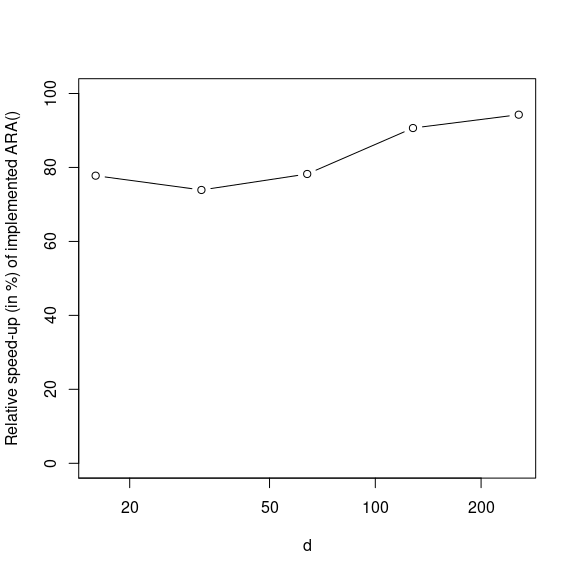### 2.3 How rearrange() acts on specific matrices To see how rearrange() actually proceeds, consider the following example. Due to trace = TRUE, the matrix is printed after each column rearrangement. A “|” and “=” indicate whether the respective column has changed or not, respectively, and the last two printed columns provide the row sums over all other columns but the current one, as well as the new updated row sums (over all columns) after the rearrangement. We see that for tol = NULL the algorithm stops after the first time $$d$$ (here: 3) consecutive rearrangements left the matrix unchanged. A <- matrix(c(1:4, 2*(1:4)-1, 2^(0:3)), ncol = 3) rearrange(A, tol = NULL, sample = FALSE, is.sorted = TRUE, trace = TRUE) ## ## [1,] 1 1 1 ## [2,] 2 3 2 ## [3,] 3 5 4 ## [4,] 4 7 8 ## | -col sum ## [1,] 4 1 1 2 6 ## [2,] 3 3 2 5 8 ## [3,] 2 5 4 9 11 ## [4,] 1 7 8 15 16 ## | -col sum ## [1,] 4 5 1 5 10 ## [2,] 3 7 2 5 12 ## [3,] 2 3 4 6 9 ## [4,] 1 1 8 9 10 ## | -col sum ## [1,] 4 5 2 9 11 ## [2,] 3 7 1 10 11 ## [3,] 2 3 4 5 9 ## [4,] 1 1 8 2 10 ## | -col sum ## [1,] 3 5 2 7 10 ## [2,] 2 7 1 8 10 ## [3,] 4 3 4 7 11 ## [4,] 1 1 8 9 10 ## = -col sum ## [1,] 3 5 2 5 10 ## [2,] 2 7 1 3 10 ## [3,] 4 3 4 8 11 ## [4,] 1 1 8 9 10 ## = -col sum ## [1,] 3 5 2 8 10 ## [2,] 2 7 1 9 10 ## [3,] 4 3 4 7 11 ## [4,] 1 1 8 2 10 ## = -col sum ## [1,] 3 5 2 7 10 ## [2,] 2 7 1 8 10 ## [3,] 4 3 4 7 11 ## [4,] 1 1 8 9 10 ##$bound
##  10
##
## $tol ##  0 ## ##$converged
##  TRUE
##
## $opt.row.sums ##  6 9 9 10 10 10 10 ## ##$X.rearranged
##      [,1] [,2] [,3]
## [1,]    3    5    2
## [2,]    2    7    1
## [3,]    4    3    4
## [4,]    1    1    8
##
## $X.rearranged.opt.row ##  2.000000 4.333333 3.666667 This is the highest possible minimal row sum and thus the optimally rearranged matrix. Let’s consider another example. B <- matrix(rep(1:3, 3), ncol = 3) rearrange(B, tol = NULL, sample = FALSE, is.sorted = TRUE, trace = TRUE) ## ## [1,] 1 1 1 ## [2,] 2 2 2 ## [3,] 3 3 3 ## | -col sum ## [1,] 3 1 1 2 5 ## [2,] 2 2 2 4 6 ## [3,] 1 3 3 6 7 ## = -col sum ## [1,] 3 1 1 4 5 ## [2,] 2 2 2 4 6 ## [3,] 1 3 3 4 7 ## = -col sum ## [1,] 3 1 1 4 5 ## [2,] 2 2 2 4 6 ## [3,] 1 3 3 4 7 ## = -col sum ## [1,] 3 1 1 2 5 ## [2,] 2 2 2 4 6 ## [3,] 1 3 3 6 7 ##$bound
##  5
##
## $tol ##  0 ## ##$converged
##  TRUE
##
## $opt.row.sums ##  5 5 5 5 ## ##$X.rearranged
##      [,1] [,2] [,3]
## [1,]    3    1    1
## [2,]    2    2    2
## [3,]    1    3    3
##
## $X.rearranged.opt.row ##  3 1 1 Here we do not reach an optimal rearrangement (6 would be a better minimal row sum, attainable via the rearrangement matrix(c(1,2,3, 2,3,1, 3,1,2), ncol = 3)). It remains an open problem how an efficient algorithm can avoid such a case. ### 2.4 Convergence Whether rearrange() converges or not depends to a large degree on the underlying sorting algorithm. We found that unstable sorting algorithms such as C’s qsort() lead to rearrange() not terminating on certain input matrices. One such example is the above matrix B, for which the 2nd/3rd column are constantly swapped after the first column being rearranged since the row sum of all others is (4,4,4) and termination then depends on how ranks are assigned to these three equal numbers. The current implementation is still not based on a stable sorting algorithm (as this would largely compromise run time) but a) is comparably fast and b) terminates on all input matrices. To see the latter, consider the following example in which we investigate the possible output matrices of rearrange() on all input matrices (obviously only for $$d = 3$$ due to run time; larger $$N$$ can be chosen but note that the effort is $$(N!)^{d-1}$$ here!). Also note that we do not actually run the code here (to save run time) ## Create a (N!)^{d-1}-list of all possible input matrices (with integer ## elements) and 1:N as first column. N <- 5 # chosen N (<= 6 due to extensive run time) x_perm <- permn(N) # N!-long list containing all possible permutations of 1:N N. <- factorial(N) mat <- vector("list", length = N.^2) cnt <- 0 for (i in 1:N.) { # double 'for' not time critical here for (j in 1:N.){ cnt <- cnt+1 mat[[cnt]] <- cbind(1:N, x_perm[[i]], x_perm[[j]]) # matrix with 1:N in first column } } ## Rearrange all these (unique) matrices N. <- factorial(N)^2 system.time(matRAlst <- lapply(1:N., function(i) { ## if(i %% (N./20) == 0) cat(round(100*i/N.),"% done!\n", sep = ""); rearrange(mat[[i]], tol = NULL, sample = FALSE)$X.rearranged
}))

## Go through all rearranged matrices, unique()ify them and count
uniqueLst <- list(matRAlst[])
freq <- c(1)
pb <- txtProgressBar(max = length(matRAlst), style = if(isatty(stdout())) 3 else 1) # setup progress bar
for(i in 2:length(matRAlst))
{
bool <- sapply(1:length(uniqueLst),
function(k) { identical(matRAlst[[i]], uniqueLst[[k]]) }) # is uniqueLst[[k]] in matRAlst?
if(any(bool)) { # matRAlst[[i]] has been observed before
ind <- min(which(bool))
freq[ind] <- freq[ind] + 1 # increase frequency
} else { # matRAlst[[i]] has not been observed before
uniqueLst <- c(uniqueLst, matRAlst[i]) # append it to uniqueLst
freq <- c(freq, 1) # append its frequency
}
## Progress
setTxtProgressBar(pb, i) # update progress bar
}
close(pb) # close progress bar
## Distribution of (final) rearranged matrices
(length(uniqueLst)/N.) * 100 # % of unique rearranged matrices
p.mat <- freq/N. # their corresponding probabilities
plot(p.mat, type = "l", xlab = "Index of unique matrices", ylab = "Probability")
## => Matrices earlier on tend to appear more often (by construction!)
## Distribution of the minimal row sums (= worst VaR estimates)
VaR.est <- sapply(matRAlst, function(x) min(rowSums(x)))
plot(VaR.est)
table(VaR.est)/length(matRAlst) # probabilities

As we can see, rearrange() terminated on all inputs. Although it generated quite a different number of rearranged matrices, the implied minimal row sums (so value-at-risk estimates) were mostly the same (8 or 9, with about 0.125% also 7).

### 2.5 A real data application

We now consider the task of computing best and worst value-at-risk (VaR) at confidence level 99% for a portfolio consisting of shares of each of the 20 constituents of the SMI from 2011-09-12 to 2012-03-28. For simplicity, the number of shares of each stock is assumed to be one over the numeric value of the stock price, so that the linearized loss is simply the sum of negative log-returns. The negative log-return of each stock is thus viewed as one loss and value-of-risk of the sum of these 20 losses is of interest. This fits precisely in the framework of the (Adaptive) Rearrangement Algorithm.

First, let’s compute the negative log-returns.

data(SMI.12)
L <- -returns(SMI.12)
n <- nrow(L)
d <- ncol(L)

Now let’s fit GPDs to each margin, that is, each SMI constituent. As threshold, we simply choose the 80% quantile for each of margins (more sophisticated methods are available).

res <- vector("list", length = d)
names(res) <- colnames(L)
for(k in seq_len(d)) { # iterate over constituents
## Determine the threshold for constituent k
L. <- L[,k]
thres <- quantile(L., probs = 0.8, names = FALSE) # threshold

## Fit a GPD to the excesses for constituent k
is.exceed <- L. > thres
p.exceed <- mean(is.exceed) # estimated threshold exceedance probability
excess <- L.[is.exceed] - thres # excesses
fit <- fit_GPD_MLE(excess) # fit a GPD to the excesses
stopifnot(fit$converged == 0) # convergence check shape <- fit$par[["shape"]] # fitted shape xi
scale <- fit$par[["scale"]] # fitted scale beta stopifnot(is.numeric(shape), is.numeric(scale), scale > 0) # sanity check ## Update res res[[k]] <- list(loss = L., excess = excess, p.exceed = p.exceed, threshold = thres, shape = shape, scale = scale) } We should also quickly assess the goodness-of-fit of the fitted GPDs if(doPDF) pdf(file = (file <- paste0("fig_worst_VaR_bounds_application_GPD_fit_check.pdf")), width = 6, height = 7) ## Graphical goodness-of-fit assessment opar <- par(omd = c(0.02, 1, 0.02, 1), mar = rep(1, 4)) layout(matrix(1:d, ncol = 4, byrow = TRUE)) # (5, 4)-layout for(k in 1:d) { x <- sort(res[[k]]$excess)
qq_plot(x, FUN = function(p)
qGPD(p, shape = res[[k]]$shape, scale = res[[k]]$scale),
axes = FALSE, frame.plot = TRUE)
mtext("Fitted quantiles", side = 1, line = 0.5, cex = 0.6)
mtext("Empirical quantiles", side = 2, line = 0.5, cex = 0.6)
op <- par(usr = c(0, 1, 0, 1))
text(1/10, 9/10, names(res)[k], cex = 0.7, adj = 0)
y <- qGPD(ppoints(length(x)), shape = res[[k]]$shape, scale = res[[k]]$scale)
R2 <- sprintf("%.4f", cor(x, y)^2) # coefficient of determination R^2
text(9/10, 1/10, substitute(R^2 == r2, list(r2 = R2)), cex = 0.7, adj = 1)
par(op)
}
layout(1) # restore layout
par(opar) # restore plot parameters
if(doPDF) dev.off()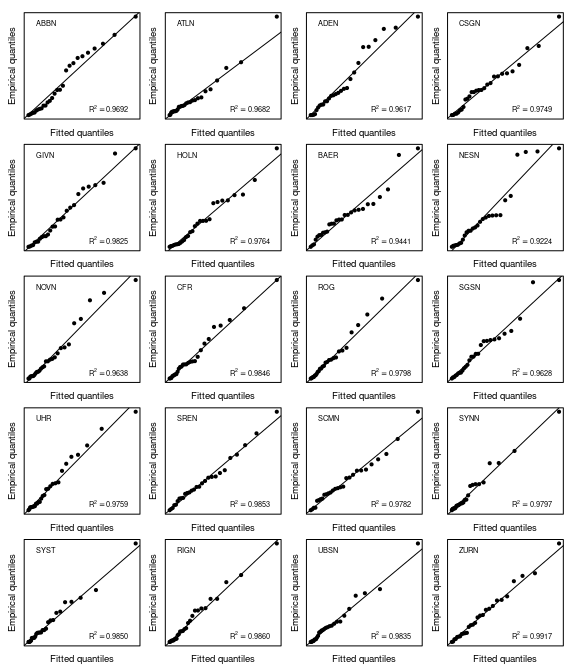We can now apply the (Adaptive) Rearrangement Algorithm to the EVT based loss distributions.

alpha <- 0.99 # confidence level
stopifnot(sapply(res, function(r) r$p.exceed) >= 1 - alpha) # bar(F)(u) >= 1-alpha <=> F(u) <= alpha ## => This was clear (as our threshold was chosen as a lower quantile than alpha ## but it is still good to check. qF <- lapply(res, function(r) { function(p) qGPDtail(p, threshold = r$threshold,
p.exceed = r$p.exceed, shape = r$shape, scale = r$scale) }) # list of quantile functions of the EVT based loss distributions set.seed(271) # set a seed (for reproducibility) res.ARA <- ARA(alpha, qF = qF) # apply ARA() stopifnot(res.ARA$converged) # check convergence
res.ARA$bounds # ARA bounds on worst VaR ## low up ## 1.229278 1.237635 res.RA <- RA(alpha, qF = qF, N = res.ARA$N.used, abstol = 0) # apply RA() (with the same N)
stopifnot(res.RA$converged) # check convergence res.RA$bounds # RA bounds on worst VaR
##      low       up
## 1.229286 1.237636

To see how the bounds on worst VaR depend on the chosen tolerance, consider the following.

## Build the input matrices for rearrange()
N <- res.ARA$N.used p.low <- alpha + (1-alpha)*(0:(N-1))/N # probability points for worst VaR (lower bound) p.up <- alpha + (1-alpha)*(1:N)/N # probability points for worst VaR (upper bound) X.low <- sapply(qF, function(qF) qF(p.low)) # input matrix (lower bound) X.up <- sapply(qF, function(qF) qF(p.up)) # input matrix (upper bound) X.up[N,] <- sapply(1:d, function(j) if(is.infinite(X.up[N,j])) qF[[j]](alpha+(1-alpha)*(1-1/(2*N))) else X.up[N,j]) ## Apply rearrange() for various tolerances (incl. NULL) tol <- seq(0, 0.5, length.out = 21) # tolerances considered res.low <- sapply(tol, function(t) rearrange(X.low, tol = t, sample = FALSE, is.sorted = TRUE)$bound)
res.low. <- rearrange(X.low, tol = NULL, sample = FALSE, is.sorted = TRUE)$bound # for tol = NULL res.up <- sapply(tol, function(t) rearrange(X.up, tol = t, sample = FALSE, is.sorted = TRUE)$bound)
res.up. <- rearrange(X.up, tol = NULL, sample = FALSE, is.sorted = TRUE)$bound # for tol = NULL ## Plot the lower and upper bound on worst VaR as a function of the chosen tol if(doPDF) pdf(file = (file <- paste0("fig_worst_VaR_bounds_application.pdf")), width = 6, height = 6) par(mar = c(5, 4+1, 4, 2) + 0.1) # increase space (for y axis label) plot(tol, res.low, type = "b", log = "y", ylim = range(res.low, res.low., res.up, res.up.), col = "royalblue3", xlab = "Relative tolerance tol of rearrange()", ylab = substitute("Bounds on"~bar(VaR)[a](L^{"+"}), list(a = alpha))) points(0, res.low., pch = 3, col = "royalblue3") # draw tol = NULL result at 0, too (as '+') lines(tol, res.up, type = "b") points(0, res.up., pch = 3) # draw tol = NULL result at 0, too (as '+') legend("topright", bty = "n", lty = rep(1,2), col = c("black", "royalblue3"), legend = c("Upper bound", "Lower bound")) if(doPDF) dev.off()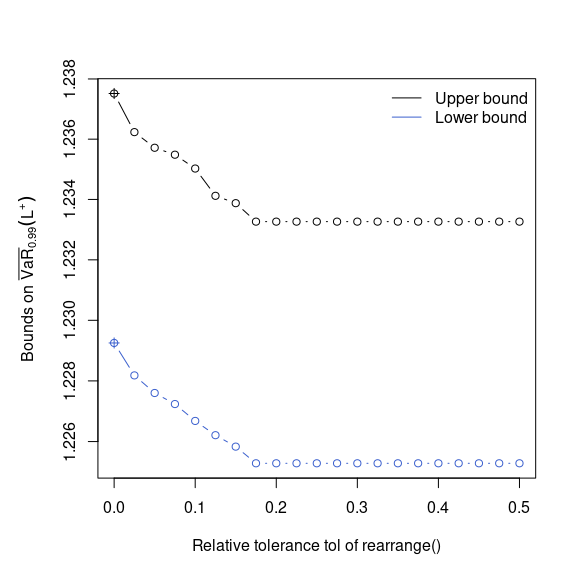As we see, the results for tol = NULL (that is, all columns oppositely ordered with respect to the sum of all others; indicated by a ‘+’) are identical to the results for tol = 0 here; the latter are typically much faster to compute. ### 2.6 Worst VaR copula samples In this section we have a quick look at a sample from the copula (of the conditional distribution of being componentwise above the confidence level) that leads to the worst value-at-risk for three given margins (Pareto, log-normal, log-gamma). ## Define parameters of the three margins th <- 2.5 # Pareto parameter m <- 10 # mean of the lognormal v <- 20 # variance of the lognormal s <- 4 # shape of the gamma underlying the loggamma r <- 5 # rate of the gamma underlying the loggamma ## Define list of marginal dfs qF <- list(qPar = function(p) (1 - p)^(-1/th) - 1, qLN = function(p) qlnorm(p, meanlog = log(m)-log(1+v/m^2)/2, sdlog = sqrt(log(1+v/m^2))), qLG = function(p) exp(qgamma(p, shape = s, rate = r))) ## Apply ARA() set.seed(271) # for reproducibility alpha <- 0.99 # confidence level wVaR <- ARA(alpha, qF = qF) # compute worst VaR (bounds) X <- wVaR[["X.rearranged"]]$up # extract rearranged matrix (upper bound)
U <- pobs(X) # compute pseudo-observations
colnames(U) <- c("U", "U", "U")
## Plot
if(doPDF)
pdf(file = (file <- paste0("fig_worst_VaR_worst_copula_sample.pdf")),
width = 6, height = 6)
cloud2(U, screen = list(z = 15, x = -60)) # default 'screen': z = 40, x = -60
if(doPDF) dev.off()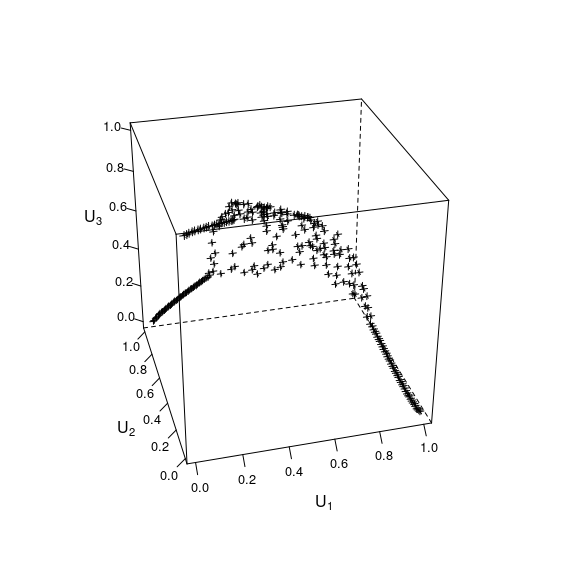We see that if one of the three components is large, the other two have to compensate for that (to keep the variance of the sum small, which is what the (A)RA tries to optimize) and so the locally singular components (strings to (1, 0, 0), (0, 1, 0), (0, 0, 1)) appear.